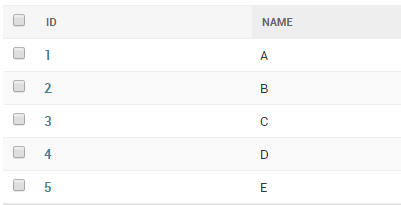# Django ORM Union, Intersection and Difference

#### ByJose Cherian

Aug 8, 2017Starting from Version 1.11 (April 2017),  Django ORM comes with Union, Intersection and Difference capabilities . While it was possible to implement this capability our own, it is nicer to have the ‘batteries included’.

Lets examine this capability using a simple model.

```class MyModel(models.Model):
name = models.CharField(max_length=1)

def __unicode__(self):
return self.name```

I have added some sample data so that we can make queriesLet’s make some queries:

```>>> from myapp.models import MyModel
>>> q1 = MyModel.objects.all()
>>> q2 = MyModel.objects.filter(name__in=('A','B'))
>>> q3 = MyModel.objects.filter(name__in=('C','D'))
>>> print q1
<QuerySet [<MyModel: A>, <MyModel: B>, <MyModel: C>, <MyModel: D>, <MyModel: E>]>
>>> print q2
<QuerySet [<MyModel: A>, <MyModel: B>]>
>>> print q3
<QuerySet [<MyModel: C>, <MyModel: D>]>```

Uses SQL’s `UNION` operator to combine the results of two or more `QuerySet`s. The `UNION` operator selects only distinct values by default. To allow duplicate values, use the `all=True` argument.

Examples:

```>>> q4 = q2.union(q3)
>>> print q4
<QuerySet [<MyModel: A>, <MyModel: B>, <MyModel: C>, <MyModel: D>]>
>>>
>>> q5 = q1.union(q2, q3) #Only distinct objects selected by default
>>> print q5
<QuerySet [<MyModel: A>, <MyModel: B>, <MyModel: C>, <MyModel: D>, <MyModel: E>]>
>>>
>>> q6 = q1.union(q2, q3, all=True) #Include duplicate objects
>>> print q6
<QuerySet [<MyModel: A>, <MyModel: B>, <MyModel: C>, <MyModel: D>, <MyModel: E>, <MyModel: A>, <MyModel: B>, <MyModel: C>, <MyModel: D>]>
```

Uses SQL’s `INTERSECT` operator to return the shared elements of two or more `QuerySet`s.

Examples:

```>>> q7 = q1.intersection(q2)
>>> print q7
<QuerySet [<MyModel: A>, <MyModel: B>]>
>>>
>>> q8 = q2.intersection(q3)
>>> print q8
<QuerySet [ ]>
>>>
>>> q9 = q1.intersection(q2, q3)
>>> print q9
<QuerySet [ ]>
>>>```

Uses SQL’s `EXCEPT` operator to keep only elements present in the `QuerySet` but not in some other `QuerySet`s.

Examples:

```>>> q10 = q1.difference(q2) #q1 - q2
>>> print q10
<QuerySet [<MyModel: C>, <MyModel: D>, <MyModel: E>]>
>>>
>>> q11 = q1.difference(q2, q3) #q1 - (q2 + q3)
>>> print q11
<QuerySet [<MyModel: E>]>
>>>
>>> q12 = q2.difference(q3) #Will return q2 as q2 and q3 are mutually exclussive
>>> print q12
<QuerySet [<MyModel: A>, <MyModel: B>]>
>>>
>>> q13 = q1.difference(q1) #q1 - q1
>>> print q13
<QuerySet [ ]>```

`union()``intersection()`, and `difference()` return model instances of the type of the first `QuerySet` even if the arguments are `QuerySet`s of other models. Passing different models works as long as the `SELECT` list is the same in all `QuerySet`s (at least the types, the names don’t matter as long as the types in the same order).

In addition, only `LIMIT``OFFSET``COUNT(*)`, and `ORDER BY` (i.e. slicing, `count()`, and `order_by()`) are allowed on the resulting `QuerySet`. Further, databases place restrictions on what operations are allowed in the combined queries. For example, most databases don’t allow `LIMIT` or `OFFSET` in the combined queries.

#### By Jose Cherian

##### 4 thoughts on “Django ORM Union, Intersection and Difference”
1. james says:

Thank you for this post. what’s the difference using union to using the | operator?

1. josecherian says:

They are similar. query1 | query2 will give a union of the two queries, but will include duplicates. For query1.uninon(query2), it returns a new queryset with only unique items by default.

2. […] my blog post on this for more […]

3. […] my blog post on this for more […]